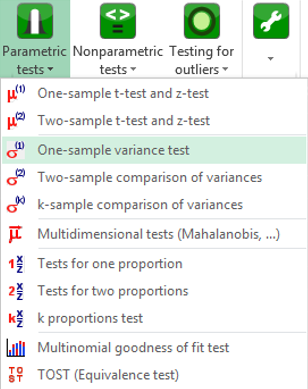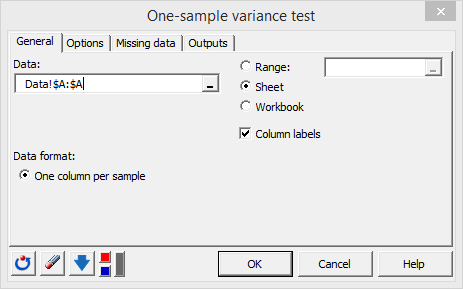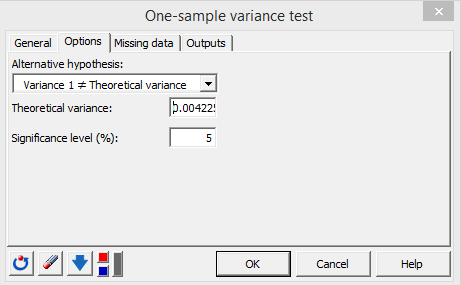# One sample variance test in Excel tutorial

2017-10-20

This tutorial will help you test the difference between an observed variance and a theoretical one, using the one sample variance test, in Excel with XLSTAT.
Not sure this is the statistical test you are looking for? Check out this guide.

## Goal of this tutorial

The goal of this tutorial is to check if an observed variance is different from a theoretical one. The test we will manipulate for this purpose can be very useful in Statistical Process Control.

## Dataset to perform a one-sample variance test

A nail factory has acquired a nail production machine. An engineer wants to test if the machine produces nails with nailhead diameter variability lower than a standard of σ = 0.065 (Standard Deviation).

Data are nail diameters measured on 50 nails produced by the machine. They can be downloaded by clicking here.

## Setting up a one-sample variance test in XLSTAT

Go over to the menu Parametric tests and select the option One-sample Variance test.In the General tab, select the data under the Data field.In the Options tab, enter the theoretical variance in the appropriate field: σ² = 0.065² = 0.004225.When everything is set, press OK. The results appear in a new spreadsheet.

## Interpreting the results of a one-sample variance test in XLSTAT

The estimated population variance is 0.0024, which is lower than the standard of 0.004225. Furthermore, the observed variance is associated to a chi-square 95% confidence interval of ] 0.0016, 0.0037 [ that does not include the standard. The subsequent p-value (0.0103) is lower than the significance threshold alpha (0.05). Thus, we can say that the machine produces nails with a head diameter variability significantly lower than the standard, with a risk of 1.03% of being wrong.The mean of nailhead diameter can also be compared to a standard using the one-sample t-test.

#### Contact our technical support team: support@xlstat.com

https://cdn.desk.com/
false
desk